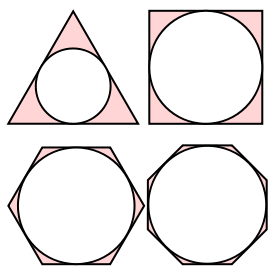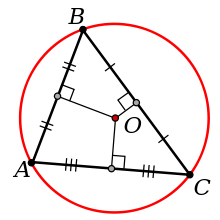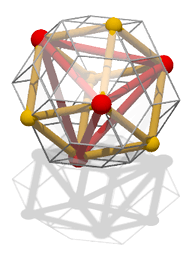# Inscribed figureAn inscribed triangle of a circleA tetrahedron (red) inscribed in a cube (yellow) which is, in turn, inscribed in a rhombic triacontahedron (grey).

In geometry, an inscribed planar shape or solid is one that is enclosed by and "fits snugly" inside another geometric shape or solid. To say that "figure F is inscribed in figure G" means precisely the same thing as "figure G is circumscribed about figure F". A circle or ellipse inscribed in a convex polygon (or a sphere or ellipsoid inscribed in a convex polyhedron) is tangent to every side or face of the outer figure (but see Inscribed sphere for semantic variants). A polygon inscribed in a circle, ellipse, or polygon (or a polyhedron inscribed in a sphere, ellipsoid, or polyhedron) has each vertex on the outer figure; if the outer figure is a polygon or polyhedron, there must be a vertex of the inscribed polygon or polyhedron on each side of the outer figure. An inscribed figure is not necessarily unique in orientation; this can easily be seen, for example, when the given outer figure is a circle, in which case a rotation of an inscribed figure gives another inscribed figure that is congruent to the original one.

Familiar examples of inscribed figures include circles inscribed in triangles or regular polygons, and triangles or regular polygons inscribed in circles. A circle inscribed in any polygon is called its incircle, in which case the polygon is said to be a tangential polygon. A polygon inscribed in a circle is said to be a cyclic polygon, and the circle is said to be its circumscribed circle or circumcircle.

The inradius or filling radius of a given outer figure is the radius of the inscribed circle or sphere, if it exists.

The definition given above assumes that the objects concerned are embedded in two- or three-dimensional Euclidean space, but can easily be generalized to higher dimensions and other metric spaces.

For an alternative usage of the term "inscribed", see the inscribed square problem, in which a square is considered to be inscribed in another figure (even a non-convex one) if all four of its vertices are on that figure.

## Properties

• Every circle has an inscribed triangle with any three given angle measures (summing of course to 180°), and every triangle can be inscribed in some circle (which is called its circumscribed circle or circumcircle).
• Every triangle has an inscribed circle, called the incircle.
• Every circle has an inscribed regular polygon of n sides, for any n≥3, and every regular polygon can be inscribed in some circle (called its circumcircle).
• Every regular polygon has an inscribed circle (called its incircle), and every circle can be inscribed in some regular polygon of n sides, for any n≥3.
• Not every polygon with more than three sides has an inscribed circle; those polygons that do are called tangential polygons. Not every polygon with more than three sides is an inscribed polygon of a circle; those polygons that are so inscribed are called cyclic polygons.
• Every triangle can be inscribed in an ellipse, called its Steiner circumellipse or simply its Steiner ellipse, whose center is the triangle's centroid.
• Every triangle has an infinitude of inscribed ellipses. One of them is a circle, and one of them is the Steiner inellipse which is tangent to the triangle at the midpoints of the sides.
• Every acute triangle has three inscribed squares. In a right triangle two of them are merged and coincide with each other, so there are only two distinct inscribed squares. An obtuse triangle has a single inscribed square, with one side coinciding with part of the triangle's longest side.
• A Reuleaux triangle, or more generally any curve of constant width, can be inscribed with any orientation inside a square of the appropriate size.